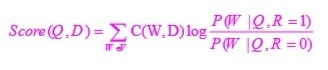# Classic Probabilistic Retrieval Model

1. [20/50 points] In the derivation of the Robertson-Sparck-Jones (RSJ) model, a multivariate Bernoulli model was used to model term presence/absence in a relevant document and a non-relevant document. Suppose, we change the model to a multinomial model (see the slide that covers both models for computing query likelihood). Using a similar independence assumption as we used in deriving RSJ, show that ranking based on probability that a document is relevant to a query Q, i.e., p(R=1|D,Q), is equivalent to ranking based on the following formula:where the sum is taken over all the words in our vocabulary (denoted by V), and c(w,D) is the count of word w in document D (i.e., how many times w occurs in D). How many parameters are there in such a retrieval model that we have to estimate?

2. [5/50 points] The retrieval function above won't work unless we can estimate all the parameters. Suppose we use the entire collection C={`D1,...,Dn`} as an approximation of the examples of non-relevant documents. Give the formula for the Maximum Likelihood estimate of p(w|Q,R=0).
3. [5/50 points] Suppose we use the query as the only example of a relevant document. Give the formula for the Maximum Likelihood estimate of p(w|Q,R=1) based on this single example of relevant document.
4. [5/50 points] One problem with the maximum likelihood estimate of p(w|Q,R=1) is that many words would have zero probability, which limits its accuracy of modeling words in relevant documents. Give the formula for smoothing this maximum likelihood estimate using fixed coefficient linear interpolation (i.e., Jelinek-Mercer) with a collection language model.
5. [15/50 points] With the two estimates you proposed, i.e., the estimate of p(w|Q,R=0) based on the collection and the estimate of p(w|Q,R=1) based on the query with smoothing, your should now have a retrieval function that can be used to compute a score for any document D and any query Q. Write down your retrieval function by plugging in the two estimates. Can your retrieval function capture the three major retrieval heuristics (i.e., TF, IDF, and document length normalization)? How?

2-[20/50 points] One way to check whether a retrieval function would over-penalize a long document is to do the following: Imagine that we duplicate a document D k times to generate a new document D' so that each term would have k times more occurrences (naturally, the new document D' is also k times longer than the original D). Intuitively, the score for D' shouldn't be less than the score of D for any query. Check if this is true for the query likelihood retrieval function with both Jelinek-Mercer smoothing and Dirichlet prior smoothing, respectively.